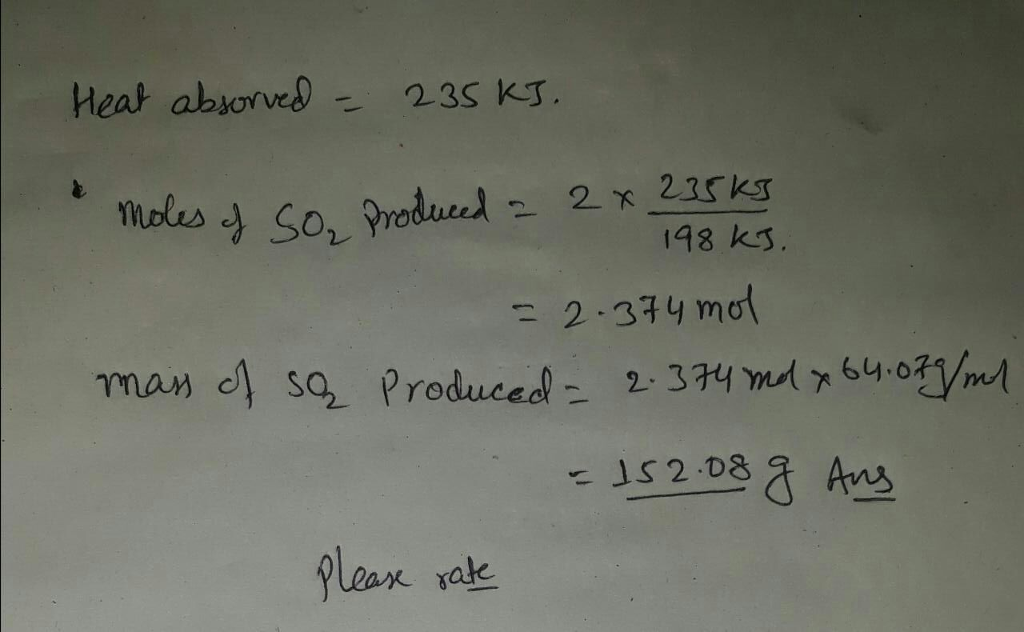# Question & Answer: If the enthalpy change for the reaction below is ΔH = 198 kJ, how many grams of sulfur dioxide…..

If the enthalpy change for the reaction below is ΔH = 198 kJ, how many grams of sulfur dioxide are produced when 235 kJ is absorbed? 2 SO3 (g) + 198 kJ –> 2 SO2 (g) + O2 (g) SO2 molar mass = 64.07 g/mol SO3 molar mass = 80.07 g/mol